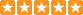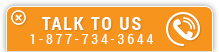# Reactance in AC Circuits - Fundamental Concepts

Course Number: E-4005
Credit: 4 PDH
Subject Matter Expert: A. Bhatia, Mechanical Engineer
Price: \$119.80 Purchase using Reward Tokens. Details10 reviews
Overview

#### In Reactance in AC Circuits - Fundamental Concepts , you'll learn ...

• What is reactance and why it occurs primarily in AC circuits
• The difference between inductive and capacitive reactance
• How to calculate impedance in AC circuits
• How to calculate apparent, true and reactive power in AC circuits

#### OverviewPreview a portion of this course before purchasing it.

Credit: 4 PDH

Length: 44 pages

Reactance is the measure of the opposition to the flow of alternating current caused by the inductance and capacitance in a circuit rather than by resistance. Steady electric currents flowing along conductors in one direction undergo opposition called electrical resistance, but no reactance. Reactance is present in addition to resistance when conductors carry alternating current. Reactance also occurs for short intervals when direct current is changing as it approaches or departs from steady flow (e.g., when switches are closed or opened).

Reactance is of two types, inductive and capacitive. Inductive reactance is associated with the varying magnetic field that surrounds a wire or a coil carrying a current. The more rapidly the current changes, the more an inductor resists it. The reactance is proportional to the frequency; a high-frequency alternating current changes more rapidly than a low-frequency one, since there are more cycles per second. Thus the higher the frequency and the larger the inductance, the harder it is for current to flow through the inductance; it meets more opposition.

Capacitive reactance is associated with the changing electric field between two conducting surfaces (plates) separated from each other by an insulating medium. The more rapidly the applied voltage changes in value, the faster the capacitor stores energy. This means that a high-frequency alternating voltage will put more current into a given capacitor than a low frequency voltage could. Thus the reactance of a capacitor goes down as the frequency increases.

This 4-hour course provides a basic overview of reactance theory, with emphasis placed on calculating inductive and capacitive reactance and impedance in AC circuits, as well as reactive and true power. Example problems with solutions are provided to aide in understanding the material.

#### Specific Knowledge or Skill Obtained

This course teaches the following specific knowledge and skills:

• The phase relationships between current and voltage in an inductor and in a capacitor
• The terms for the opposition an inductor and a capacitor offer to AC
• The formulas for inductive and capacitive reactances
• The effects of a change in frequency on inductive and capacitive reactances
• The effects of a change in inductance on capacitance and vice versa
• How to compute total reactance (X) in a series circuit and determine whether the total reactance is capacitive or inductive
• The formula for total reactance and impedance
• The Ohm's law formulas used to determine voltage and current in an ac circuit
• Definition of "true power", "reactive power", and "apparent power"
• The definition of and the formula for power factor

#### Certificate of Completion

You will be able to immediately print a certificate of completion after passing a multiple-choice quiz consisting of 20 questions. PDH credits are not awarded until the course is completed and quiz is passed.

Board Acceptance
 This course is applicable to professional engineers in: Alabama (P.E.) Alaska (P.E.) Arkansas (P.E.) Delaware (P.E.) Florida (P.E. Area of Practice) Georgia (P.E.) Idaho (P.E.) Illinois (P.E.) Illinois (S.E.) Indiana (P.E.) Iowa (P.E.) Kansas (P.E.) Kentucky (P.E.) Louisiana (P.E.) Maine (P.E.) Maryland (P.E.) Michigan (P.E.) Minnesota (P.E.) Mississippi (P.E.) Missouri (P.E.) Montana (P.E.) Nebraska (P.E.) Nevada (P.E.) New Hampshire (P.E.) New Jersey (P.E.) New Mexico (P.E.) New York (P.E.) North Carolina (P.E.) North Dakota (P.E.) Ohio (P.E. Self-Paced) Oklahoma (P.E.) Oregon (P.E.) Pennsylvania (P.E.) South Carolina (P.E.) South Dakota (P.E.) Tennessee (P.E.) Texas (P.E.) Utah (P.E.) Vermont (P.E.) Virginia (P.E.) West Virginia (P.E.) Wisconsin (P.E.) Wyoming (P.E.)
Reviews (10)
More DetailsPreview a portion of this course before purchasing it.

Credit: 4 PDH

Length: 44 pages